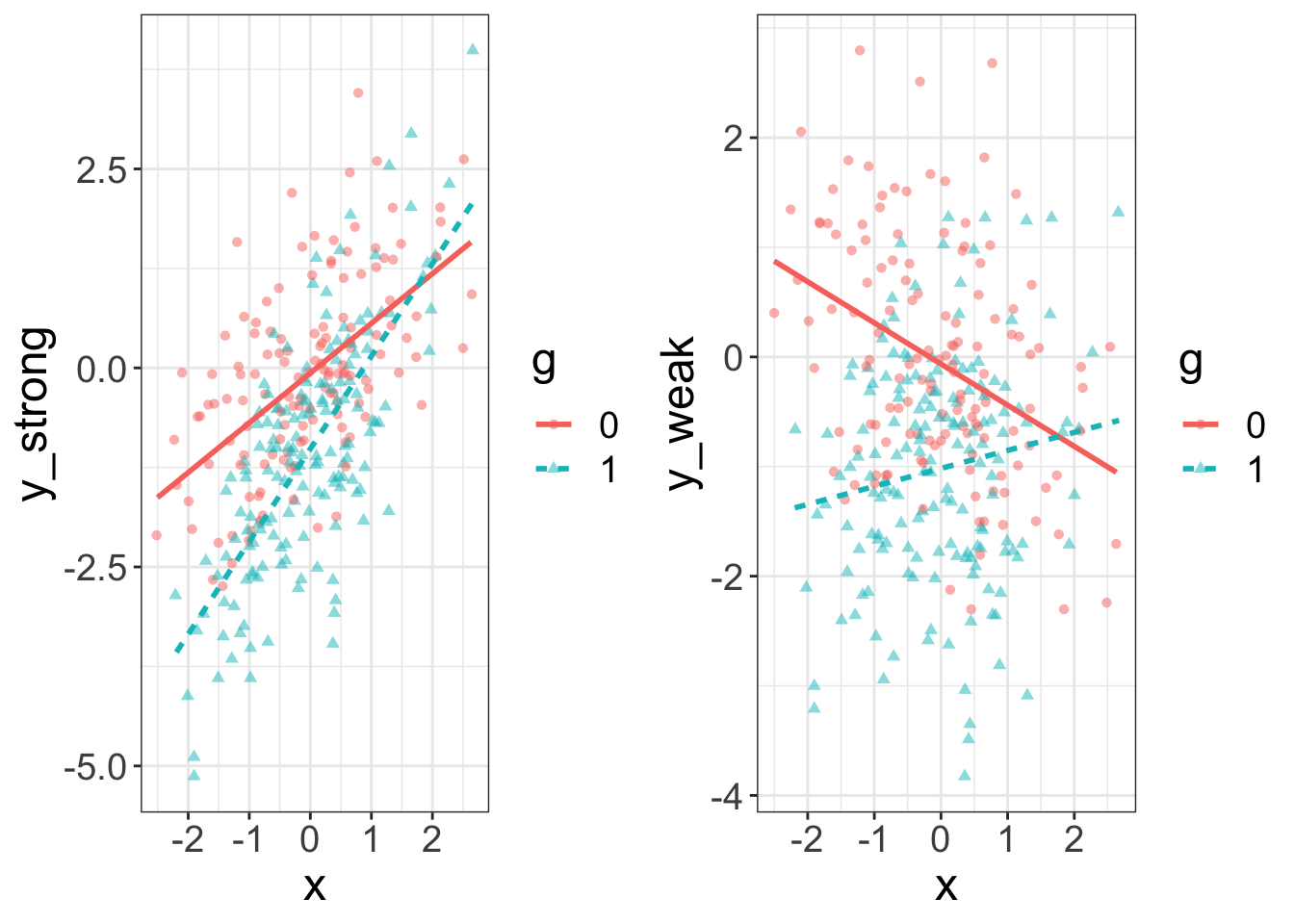I recently received an email from a student. The cool thing about answering questions is it gives you a chance to learn something you knew but didn’t know you knew (see my response to Questions 3/4 below).

Here’s the email (her email is italicized and my responses are bolded):

I am running the following model ->

y~b0+(b1)x1+(b2)x2+(b3)x1*x2

x1 is continuous and x2 is categorical (gender, two levels OR race, three levels)
I’m interested in the interaction term.

I have been using the summary function to get the estimate and p-value for the interaction term. My questions are as follows:
Q2: Does the magnitude of standardized beta for an interaction term really mean anything interesting when the moderator is categorical? In my head it means that for every SD increase in the interaction term the DV changes by “beta” SDs. Correct? The sign of it clearly means something (whether the relationship b/w y and x1 gets more pos or more neg when you change groups), but the actual value does not seem meaningful. Is that right?

Let’s do some math:

y = b0 + b1*x + b2*group + b3*group*x

Let’s assume we’re the referent group (i.e., group = 0):

y = b0 + b1*x + b2*(0) + b3*(0)*x
y = b0 + b1*x

And for the other group:

y = b0 + b1*x + b2*(1) + b3*(1)*x
y = (b0 + b2) + (b2 + b3)*x

So, b2 is the difference between groups in the intercept, and b3 is the difference between groups in the slope. If it’s a standardized beta, b3 represents the difference in the amount of standard deviations y changes for a standard deviation change in x (relative to the referent group). Make sense?

Q3: Relatedly, is it better to just report the semi-partial R-squared for the interaction term? What is the most informative estimate here?
That’s a tough question to answer. I’d probably be inclined to report the standardized beta and interpret it correctly in the paper (i.e., as a difference in slopes). The semi-partial R squared isn’t as standardized as you would think. Suppose you have two models that have slopes that deviate by exactly the same amount (i.e., the interaction betas are equivalent). Also suppose the first model has a large “main effect” (e.g., there’s generally a positive slope for x), and suppose the second model has no main effect (i.e., there’s a crossover interaction, producing an average main effect of zero). The semi-partial will partition the explained variance. In the first model (the one with a large main effect), much of that variance is going to be sucked up by the main effect and so the interaction effect is going to appear quite small. On the other hand, almost all of the explained variance for the second model is going to be given to the interaction. Remember, these two models have identical sized interactions, yet the semi partials are going to be very different. Make sense?

Q4: Can the magnitude of a standardized beta for an interaction term be above 1? This is what the internet seems to say but it is confusing. Some of my betas from summary(mod) are >1, which is why I initially went down this rabbit hole.

I suppose it could. Because b3 is the difference in slopes, you could have a strongly negative slope for group 1 (e.g., b1 = -0.7) and a strongly positive slope for group 2 (e.g., +0.8). To make this happen, your interaction term must be greater than one (it would be 1.1 in this case).

My response makes sense, but I figured I’d actually simulate this to make sure it makes sense.

  ## simulate same random normal data for both conditions
n = 300
set.seed(1212)
x = rnorm(n)
y = rnorm(n)
g = sample(c(1,0), size=n, replace=T)

## one model with a strong main effect
y_strong = .7*x -1*g + .3*x*g + y

## one model with a weak negative main effect, but identical sized interaction term
y_weak =  - .3*x -1*g + .3*x*g + y

## combined into data frame
d = data.frame(x=x, y=y, g=g, y_strong=y_strong, y_weak=y_weak)
d$g = as.factor(d$g)

Now let’s visualize them:

## visualize them
require(flexplot)
a = flexplot(y_strong~x + g, data=d, method="lm")
b = flexplot(y_weak~x + g, data=d, method="lm")
cowplot::plot_grid(a,b)If we look at the models, the coefficients for the interaction are identical (as they should be):

mod_strong = lm(y_strong~x*g, data=d)
mod_weak = lm(y_weak~x*g, data=d)
coef(mod_strong)
## (Intercept)           x          g1        x:g1
## -0.06460258  0.62464595 -0.95230473  0.53946943
coef(mod_weak)
## (Intercept)           x          g1        x:g1
## -0.06460258 -0.37535405 -0.95230473  0.53946943

Now, let’s look at the semi-partials:

estimates(mod_strong)$semi.p ## Note: I am not reporting the semi-partial R squared for the main effects because an interaction is present. To obtain main effect sizes, drop the interaction from your model. ## Note: You didn't choose to plot x so I am inputting the median ## x g x:g ## 0.36870573 0.11421068 0.03484555 estimates(mod_weak)$semi.p
## Note: I am not reporting the semi-partial R squared for the main effects because an interaction is present. To obtain main effect sizes, drop the interaction from your model.
##
##
## Note: You didn't choose to plot x so I am inputting the median
##          x          g        x:g
## 0.01169347 0.17879960 0.05455156

Notice that the semi-partials are different: the one with the weak effect is much larger. Also, proportionally, the semi-partial for the strong main effect model is 0.03/0.518 = 0.058, while the proportion for the semi-partial of the weak main effect model is 0.054/0.245 = 0.22. In other words, the semi-partial for the model with a weak main effect seems larger than the one with the strong main effect. Once again, this is because the semi-p assigns chunks of variance explained to each component. Though in absolute value the interactions are identical, in relative value the one with the weak main effect seems much stronger.